# Going Full Circle

#### Lesson Overview

This lesson completes our review of basic geometry. It primarily deals with theorems regarding circles and lines which intersect them. I include here links to an old but good childhood toy called Spirograph. Check out these applets.

### Arcs, Chords, and their Measure; picture angles

We defined arcs and chords in lesson 8. Be sure to review the meaning of minor arc, major arc, and semicircle, as well as sector, segment, and annulus. We also defined inscribed and central angles in lesson 3.

Although all circles are similar, note that circles are congruent if and only if they have the same radius. We extend this concept to arcs and chords in congruent circles being congruent if and only if they have the same measure. Several additional properties are summaried in the following theorem about chords and the circle's center. It can be proved using the properties of the altitude, median, and perpendicular bisector of an isosceles triangle.

 The perpendicular bisector of a chord of a circle contains the center of the circle. The line containing the center of a circle and perpendicular to a chord bisects the chord. The bisector of the central angle of a chord perpendicularly bisects the chord. The line containing the circle's center and the midpoint of a chord bisects the chord's central angle.

We repeat here the relationship between the measure of an inscribed angle and corresponding central angle.

 An inscribed angle is half the measure of the central angle intercepting the same arc.

Since a semicircle is 180° the following is also true.

 An angle inscribed in a semicircle is right.

Of course,
 If two inscribed angles intercept the same arc, then they have the same measure.

If you know the measure of a central angle (and radius), you can calculate the arc length of its corresponding arc as the proportion between this measure over 360° equal to this unknown arc length over the circumference.

Example: Suppose you have a 60° arc in a circle with radius 6 m. Find its arc length A.
Solution: 60° is 1/6 of a circle, so the arc length is 1/6 the circumference: C=12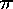or A=2.

Our textbook motivates this further by referring to the picture angle or field of vision of a camera lens. A normal lens has a picture angle of about 62°, whereas a wide angle lens may be as large as 118°, and a telephoto lens might be as small as 18°. It continues by using an inscribed angle to relate picture angle, and in the next section, the corresponding central angle and trigonometry to find the minimal distance from the building.

Example: A camera has a 56° picture angle. Where can a person stand so that the front of a 60' building just fills the picture.
Solution: Let the ends of the front of the building be points A and B. These form a chord of a circle O. All points P which form a 56° inscribed angle (and are in front of the building) are places the person could stand. The corresponding central angle would be 112°. If the person stands directly in front of the building an isosceles triangle is formed which we can bisect and have a 28°–62°–right triangle. 30/d=tan 28° or d=30/tan 28°=56.4'.

One can construct the center of the circle by locating the point of intersection of the bisectors of two chords of the circle (perpendicular bisector method). Using right angles and the corresponding semicircles is another way (right angle method). Although draftsmen (and draftswomen) often use a T-square or square (ell), for drawing purposes, the corner of a book or index card will suffice. Be able to construct or draw the center of a circle when only given an arc.

### Chords and Secants Form Angles

Although the term secant and tangent were defined as trigonometric functions in lesson 13, in this lesson we will be use them to refer to special geometric lines related to a circle.

 A secant is the line containing a chord. It thus intersects a circle at the chord's two endpoints.

 The measure of an angle formed by two intersecting chords is one-half the sum of the measures of the arcs intercepted by it and its vertical angles.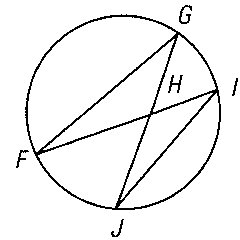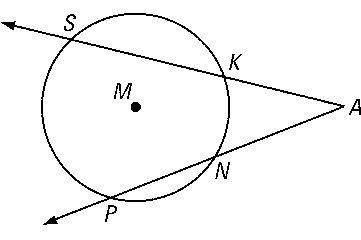The measure of an angle formed by two secants intersecting outside a circle is half the difference of the arcs intercepted by the angle.

### Tangents and Angles so Formed

 A tangent (to a circle) is a line in the plane of the circle which intersecting the circle at one and only one point.

The point of tangency is the point where the line and circle intersect. The word tangent is also used to describe other situations where circle or spheres intersect (internally tangent, externally tangent, etc). A common tangent is a single line which is tangent to more than one object.

 A line is perpendicular to the radius at the radius endpoint on a circle if and only if it is a tangent line.Example: A common application of this last theorem is to find the distance to the horizon from the top of a building, tower, or plane. Using the value 1260', which could apply to any of these, find the desired result.
Solution: Assume the earth is a perfect sphere with r=3960 miles. 1260'=¼ miles or 0.25 miles. Using the Pythagorean theorem with the right angle at the horizon, we find the missing side is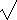(3960.252-39602)=44.50 miles!

 The measure of an angle formed by a tangent and a chord is half the measure of its intercepted arc.

 The measure of the angle between two tangents (or between a tangent and a secant) is the difference of the intercepted arcs.

### Lengths of Chords, Secants, and Tangents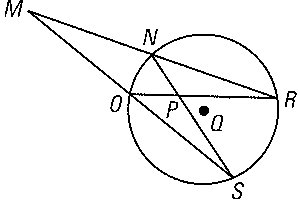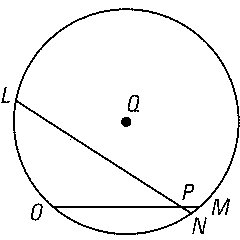Let one secant intersect a circle at A and B and another secant intersect the circle at C and D. If the point of intersection is P, then AP•BP=CP•DP.

Euclid's proof works whether P is inside or outside the circle! Steiner called the product used above the power of the point P for the circle.

 The power of point P for a circle is the square of the length of a segment tangent to the circle from P.

### Isoperimetric

Isoperimetric means having equal perimeters.

 Given two different figures with the same perimeter (circumference), the circle has the greatest area.

It follows that:
 Of different figures with the same area, the circle has the smallest perimeter.

This fact sets an upper bound on a figure's area given its perimeter. Specifically, if a figure has perimeter P, then its area A must be less than or equal to P2/(4).

Analogous 3D versions of these theorems also holds.

 Given two different solids with the same surface area, the sphere has the greatest volume.

 Of different solids with the same volume, the sphere has the smallest surface area.

Sometimes a large surface area is important. The recent history of the development of the catalytic converter is a case in point. Several technological factors had to be overcome, such as, get lead out of gasoline, find something which could be economically manufactured with a large surface area which would withstand high temperature, etc. Thus inside the catalytic converter you find a honeycomb of ceramic material coated with a catalyst. Usually there are two reaction areas, a reduction area using platinum or rhodium as catalyst and an oxidation area using platinum or palladium. All these metals are very expensive. Sponges (living, dead, or synthetic) are another example where a large surface area is desired.